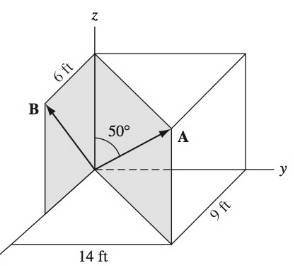# Use the dot product to find the angle between the position vectors A and B.### International Edition---engineerin...

4th Edition
Andrew Pytel And Jaan Kiusalaas
Publisher: CENGAGE L
ISBN: 9781305501607

#### Solutions

Chapter
Section### International Edition---engineerin...

4th Edition
Andrew Pytel And Jaan Kiusalaas
Publisher: CENGAGE L
ISBN: 9781305501607
Chapter 1, Problem 1.62P
Textbook Problem
305 views

## Use the dot product to find the angle between the position vectors A and B.To determine

The value of angle between the position vectors A and B.

### Explanation of Solution

Given Information:

The formula for dot product is

AB=|A||B|cos(θ)A.B=AxBx+AyBycos(θ)=AxBx+AyBy|A||B|θ=cos1( A x B x + A y B y |A||B|)

Calculation:

So we have to find OC

|OC|= ( 14 )2+ ( 9 )2|OC|=16.64fttan500=ACOCAC=OCtan500AC=16.64(tan 400)AC=19.83ftOA=9i+14j+19

### Still sussing out bartleby?

Check out a sample textbook solution.

See a sample solution

#### The Solution to Your Study Problems

Bartleby provides explanations to thousands of textbook problems written by our experts, many with advanced degrees!

Get Started

Find more solutions based on key concepts
What is the purpose of a clearance plane in CNC programming and milling?

Precision Machining Technology (MindTap Course List)

For Example 3 in Table 18.8, verify that the given solution satisfies the governing differential equation and t...

Engineering Fundamentals: An Introduction to Engineering (MindTap Course List)

What is a negative feedback loop? How is it used to keep a project in control?

Principles of Information Security (MindTap Course List)

Define and explain the different types of distribution transparency.

Database Systems: Design, Implementation, & Management

Use A992 steal and. select a W shape. a. Use LRFD. b. Use ASD.

Steel Design (Activate Learning with these NEW titles from Engineering!)